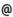## "Babes-Bolyai" University of Cluj-Napoca Faculty of Mathematics and Computer Science

 Real analysis (1)
 Code Semes-ter Hours: C+S+L Credits Type Section MT001 4 2+2+0 6 compulsory Matematică MT001 4 2+2+0 6 compulsory Matematică-Informatică MT001 4 2+2+0 6 compulsory Matematici aplicate
 Teaching Staff in Charge
 Assoc.Prof. ANISIU Valeriu, Ph.D., anisiumath.ubbcluj.ro
 Asist. NECHITA Veronica Oana, veromath.ubbcluj.ro
 Aims Basic facts on general topology and measure theory with applications in calculus.
 Content 1. ELEMENTS OF GENERAL TOPOLOGY: topological spaces, neighbourhoods, closure, interior, boundary. Basis and subbasis, topology generated by a family of subsets, subspaces, the product of two topological spaces. Convergence and continuity, Heine's theorem, sequential characterization of the closure. The separation axioms (T1 and T2). The topology of metric and semimetric spaces, completeness. Compact, sequentially compact, and precompact spaces, Hausdorff's theorem. Connected spaces, normal spaces and Tietze's theorem. 2. MEASURE THEORY: Algebras and sigma-algebras. Abstract measure, sigma-additivity. Exterior measures, measurable sets with respect to exterior measures, Caratheodory's theorem. The Lebesgue measure in Euclidean spaces, regular measures. 3. LEBESGUE INTEGRAL: Measurable and simple functions, arithmetic operations and limits, the approximation theorem. The construction of the Lebesgue integral. The monotone convergence theorem and the dominated convergence theorem. Application in calculus (integrals with parameters). Relation with the Riemann integral (proper and improper).
 References 1. V. ANISIU: Topologie si teoria masurii. Universitatea "Babes-Bolyai", Cluj-Napoca, 1995. 2. C. CRACIUN: Lectii de analiza matematica. Universitatea Bucuresti, 1982. 3. C. CRACIUN: Exercitii si probleme de analiza matematica. Universitatea Bucuresti, 1984. 4. B. GOSTIAUX: Exercises de mathematiques speciales, Tome 2, Presse Universitaire de France, 1997. 5. P. KREE: Integration et theorie de la mesure. Une approche geometrique. Ellipses, Paris, 1997. 6. W. RUDIN: Real and complex analysis, McGraw Hill, New York, 1988 (exista traducere in limba romana)
 Assessment Tests (30%) and final exam (70%).